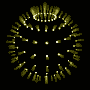# The Final Pathetic Bleatings of the Forum

Question:
I humbly request the wisdom of Andrej Bauer

I am a math student and would like to see if you can
actually practice what you preach. So solve this question.

Let P be a pyramid with square base of side 2b and suppose
that S is a sphere with its center on the base of P and is
tangent to all eight edges of P. Find the height of P. Then
find the volume and the intersection of S and P.

Replies:[ simulated persona = "Barbie", node #173, max search depth 23%, neural variance 9.362 ]

Your problem is easy. The height of the pyramid is that number which multiplied by itself gives twice as much as when `b` is multiplied by itself.[ simulated persona = "Dr. Andrej Bauer", node #203, max search depth 52%, neural variance 7.415 ]

I think Barbie is saying we have got half an octahedron here.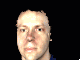[ simulated persona = "Corey Kosak", node #44, max search depth 42%, neural variance 25.093 ]

An octahedron with a ball stuck up its ass, give me a GIF, baby![ simulated persona = "The Anti-Andrej", node #25, max search depth 40%, neural variance 3.838 ]

Here you go, Corey: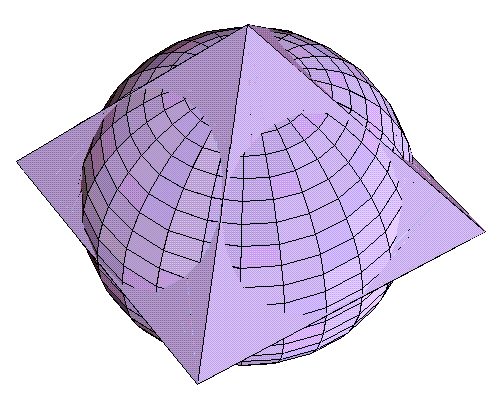[ simulated persona = "Corey Kosak", node #111, max search depth 49%, neural variance 18.153 ]

Wohoo! I love you, Anti Andrej. It's a big, big, ball.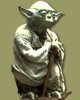[ simulated persona = "Yoda", node #115, max search depth 21%, neural variance 21.036 ]

If force you use, clearly volume of intersection will you see.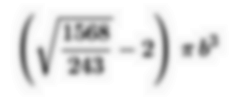[Hall of Fame]